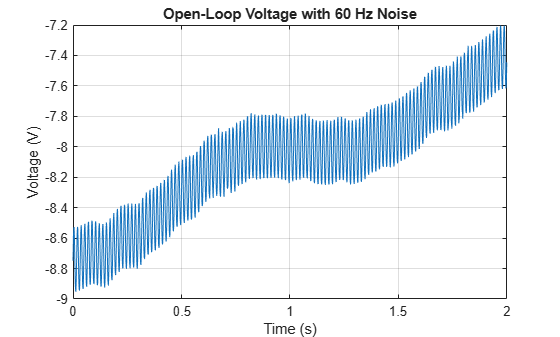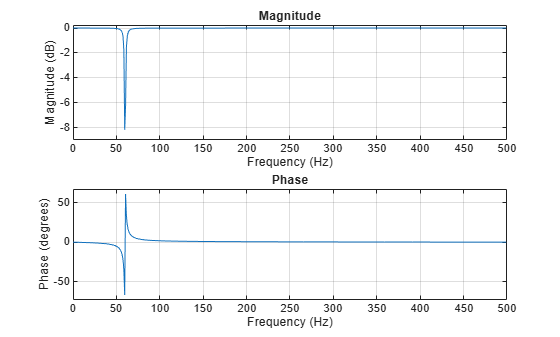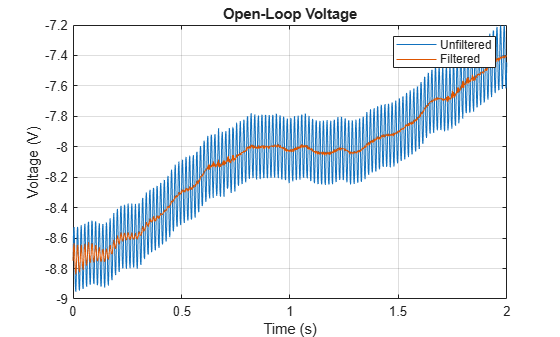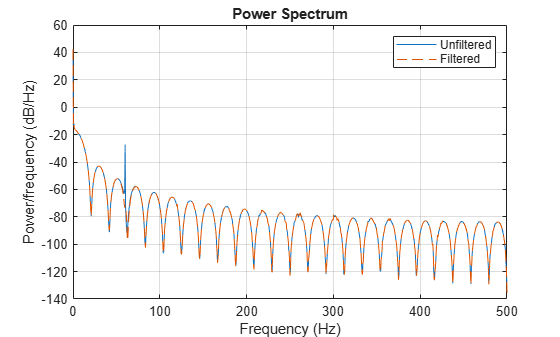Documentation

## Remove the 60 Hz Hum from a Signal

Alternating current in the United States and several other countries oscillates at a frequency of 60 Hz. Those oscillations often corrupt measurements and have to be subtracted.

Study the open-loop voltage across the input of an analog instrument in the presence of 60 Hz power-line noise. The voltage is sampled at 1 kHz.

```load openloop60hertz, openLoop = openLoopVoltage; Fs = 1000; t = (0:length(openLoop)-1)/Fs; plot(t,openLoop) ylabel('Voltage (V)') xlabel('Time (s)') title('Open-Loop Voltage with 60 Hz Noise') grid```Eliminate the 60 Hz noise with a Butterworth notch filter. Use `designfilt` to design it. The width of the notch is defined by the 59 to 61 Hz frequency interval. The filter removes at least half the power of the frequency components lying in that range.

```d = designfilt('bandstopiir','FilterOrder',2, ... 'HalfPowerFrequency1',59,'HalfPowerFrequency2',61, ... 'DesignMethod','butter','SampleRate',Fs);```

Plot the frequency response of the filter. Note that this notch filter provides up to 45 dB of attenuation.

`fvtool(d,'Fs',Fs)`Filter the signal with `filtfilt` to compensate for filter delay. Note how the oscillations decrease significantly.

```buttLoop = filtfilt(d,openLoop); plot(t,openLoop,t,buttLoop) ylabel('Voltage (V)') xlabel('Time (s)') title('Open-Loop Voltage') legend('Unfiltered','Filtered') grid```Use the periodogram to see that the "spike" at 60 Hz has been eliminated.

```[popen,fopen] = periodogram(openLoop,[],[],Fs); [pbutt,fbutt] = periodogram(buttLoop,[],[],Fs); plot(fopen,20*log10(abs(popen)),fbutt,20*log10(abs(pbutt)),'--') ylabel('Power/frequency (dB/Hz)') xlabel('Frequency (Hz)') title('Power Spectrum') legend('Unfiltered','Filtered') grid```